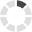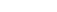### Site InformationLoading... Please wait...

# ECET 330 ECET/330 ECET330 QUIZ 5

\$30.00

## Product Description

ECET 330 ECET330 QUIZ 5

1. (TCO 5) For the SUBA #\$66 in the following code, the ALU performs:

LDAA     #\$66
SUBA     #\$99

A = __________

2.

(TCO 5) Find the value in Register A after the execution of the following code:

LDAA     #\$37
ORAA    #\$F0

A = __________

3.

(TCO 5) Find the value in Register A after the execution of the following code:

LDAA     #\$66
COMA                   ;complement A
ADDA     #01

A = __________

4.

(TCO 5) Which bit is used to indicate the sign of a 16-bit signed number?

5.

(TCO 5) Which of the following is +9 for an eight-bit signed number?

6.

(TCO 5) Find the value in Register A after the execution of the following code:

LDAA     #\$56
SUBA     #\$93

A = __________

7.

(TCO 5) Find the value in Register A after the execution of the following code:

LDAA     #\$37
ORAA    #\$F0

A = __________

8.

(TCO 5) Find the value in Register A after the execution of the following code:

LDAA     #\$FF
COMA                   ;complement A
ADDA #1

A = __________

9.

(TCO 5) Which bit is used to indicate the sign of an eight-bit signed number?

10. (TCO 5) Which of the following is +9 for an eight-bit signed number?

11. (TCO 5) Find the value in Register A after the execution of the following code:

LDAA     #\$99
SUBA     #\$66

A = __________

12. (TCO 5) Find the value in Register A after the execution of the following code:

LDAA     #\$37
ORAA    #\$F0

A = __________

13. (TCO 5) Find the value in Register A after the execution of the following code:

CLRA                                      ;Clear A
COMA                   ;complement A
LSLA

A = __________

14. (TCO 5) Which bit is used to indicate the sign of a 16-bit signed number?

15. (TCO 5) Which of the following is +9 for an eight-bit signed number?

16. (TCO 5) Find the value in Register A after the execution of the following code:

LDAA     #\$32
SUBA     #\$3E

A = __________

17. (TCO 5) Find the value in Register A after the execution of the following code:

LDAA     #\$37
ANDA    #\$0F

A = __________

18. (TCO 5) Find the value in Register A after the execution of the following code:

LDAA     #0
COMA                   ;complement A
ADDA #1

A = __________

19. (TCO 5) Which bit is used to indicate the sign of an eight-bit signed number?

20. (TCO 5) Which of the following is +9 for an eight-bit signed number?

21. (TCO 5) Find the value in Register A after the execution of the following code:

LDAA     #\$99
SUBA     #\$66

A = __________

22. (TCO 5) Find the value in Register A after the execution of the following code:

LDAA     #\$37
ORAA    #\$F0

A = __________

23. (TCO 5) Find the value in Register A after the execution of the following code:

CLRA                                      ;Clear A
COMA                   ;complement A
ADDA #01

A = __________

24. (TCO 5) For a 16-bit signed number, we can use a maximum of __________ bits for the non-sign portion (magnitude) of the signed number.

25. (TCO 5) The largest eight-bit positive signed number that we can have is

26. (TCO 5) For the SUBA #\$66 in the following code, the ALU performs:

LDAA     #\$99
SUBA     #\$66

A = ___________

27. (TCO 5) Find the value in Register A after the execution of the following code:

LDAA     #\$55
EORA     #\$FF

A = __________

28. (TCO 5) Find the value in Register A after the execution of the following code:

CLRA                                      ;Clear A
COMA                   ;complement A
ADDA #01

A = __________

29. (TCO 5) For a 16-bit signed number, we can use a maximum of __________ bits for the non-sign portion (magnitude) of the signed number.

30. (TCO 5) Which of the following is +5 for an eight-bit signed number?

31. (TCO 5) Find the value in Register A after the execution of the following code:

LDAA     #\$99
SUBA     #\$66

A = __________

32. (TCO 5) Find the value in Register A after the execution of the following code:

LDAA     #\$AA
EORA     #\$FF

A = __________

33. (TCO 5) Find the value in Register A after the execution of the following code:

LDAA     #0
COMA                   ;complement A
ADDA #1

A = __________

34. (TCO 5) Which bit is used to indicate the sign of a 16-bit signed number?

35. (TCO 5) Which of the following is -5 for an eight-bit signed number?

36. (TCO 5) For the SUBA #\$66 in the following code, the ALU performs:

LDAA     #\$99
SUBA     #\$66

A = ___________

37. TCO 5) Find the value in Register A after the execution of the following code:

LDAA     #\$AA
EORA     #\$FF

A = __________

38. (TCO 5) Find the value in Register A after the execution of the following code:

LDAA     #0
COMA                   ;complement A
ADDA #1

A = __________

39. (TCO 5) Which flag is used to see if the signed data are correctly added together?

40. (TCO 5) Which of the following is +9 for an eight-bit signed number?

## Customers also viewed

• \$24.99• \$35.00• \$18.00• \$18.00• \$35.00## Related Products

• ECET 330 ECET/330 ECET330 QUIZ 1 \$30.00• ECET 330 ECET/330 ECET330 QUIZ 6 \$40.00• ECET 330 ECET330 Week 8 Final Exam \$24.99• ECET 330 Week 1 Homework \$18.00• ECET 330 Week 5 Homework \$18.00Click the button below to add the ECET 330 ECET/330 ECET330 QUIZ 5 to your wish list.